# Comparison between Investment or Annuity

In this article we will learn how to calculate the investment value for future or annuity for the present value by using the functions of Microsoft Excel.

When we made investment, the amount paid is entered in negative value so as to get the accurate result.

Annuity is the return we get and is written in positive because in future we have to pay interest amount along with principal amount.

INVESTMENT:

Let’s take an example and understand:

We want to invest \$1000 every year, on the 10% rate of interest and for 10 years, then how much total value we will get after 10 years?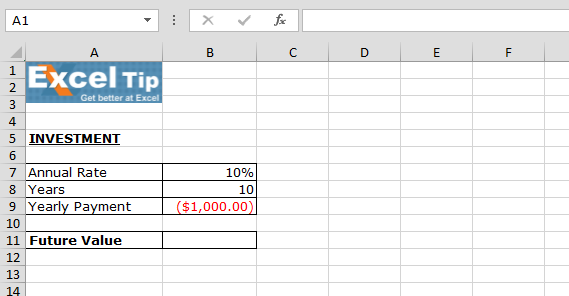To calculate Future Value follow below given steps:-

• Enter the function in cell B11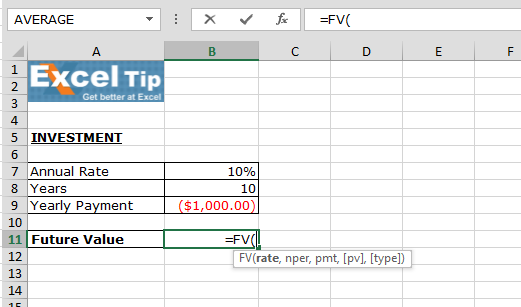• Select annual rate
• Select number of years
• And then select yearly payment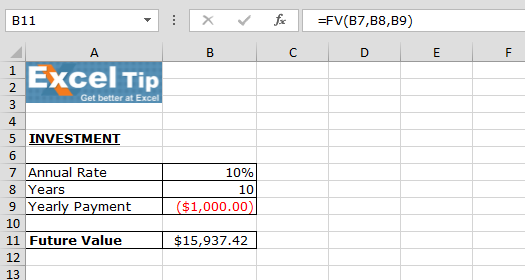As per the calculation, if we pay \$1,000 in a year it means we have to pay \$10,000, after 10 years we will get \$15,937.42.

Note: In this function last two arguments are optional, if we omit it, then function assumes that payments are due at the end of the period.

ANNUITY:

We need to purchase annuity for 12 years with the amount \$200 on monthly basis and rate of interest is 8%, how much does the annuity cost?

• Enter the Present value’s function in cell B11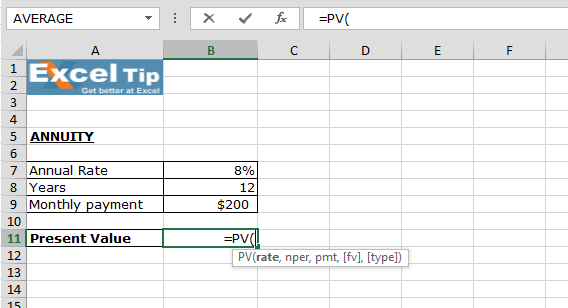• Select rate of interest and divide by 12 to calculate the monthly rate of interest
• Then select years and multiply by 12, to calculate the total value
• Select monthly payment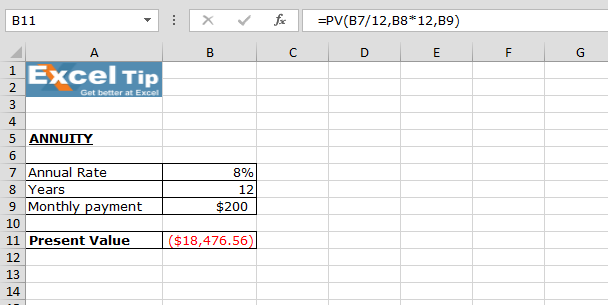As per the result, we have to pay \$18,476.56 till the 12 years or we can do onetime payment.

Note: The last two arguments are optional. If omitted, Fv = 0 (no future value). If Type is omitted, it is assumed that payments are due at the end of the period. This annuity does not take into account life expectancy, inflation etc